### ROWS Function Examples – Excel & Google Sheets

This Tutorial demonstrates how to use the Excel ROWS Function in Excel to count the number of rows.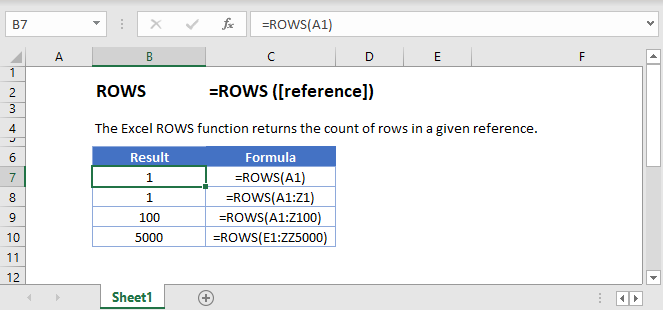## ROWS Function Description:

The ROWS Function Returns the number of rows in an array.

To use the ROWS Excel Worksheet Function, select a cell and type: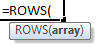(Notice how the formula inputs appear)

## ROW function Syntax and inputs:

array – Count the number of rows in this array.

Use the ROWS Function to calculate the number of columns in a range of cells.

## ROWS Function

The ROWS Function returns the number of rows in a given cell reference.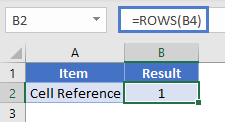## ROWS Function – Cell Range

You can also enter a cell range and it will return the total number of rows in it.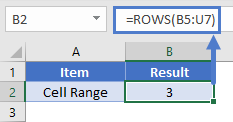## ROWS Function – Array Constant

You can also count the number of rows in a given array constant.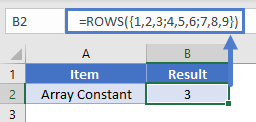## ROWS Function in Google Sheets

The ROWS Function works exactly the same in Google Sheets as in Excel: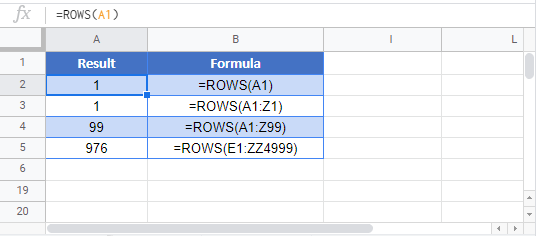## Excel Practice Worksheet

Practice Excel functions and formulas with our 100% free practice worksheets!